Posted by James

Minimum Number Of Decreasing Subsequence Partitions ONLINE JUDGE ⭐⭐⭐
Rose Garden ONLINE JUDGE
Fill 2D Array ONLINE JUDGE
Pick Up Coupons ONLINE JUDGE
Water Plants ⭐
Compare Strings ⭐
Largest Subarray ⭐
Maximum Area Serving Cake ⭐
Key Formatting ⭐

#### Google OA : Minimum Number Of Decreasing Subsequence Partitions

Given an int array of length n. Split it into strictly decreasing subsequences. Output the minimum number of decreasing subsequences you can get by splitting the array...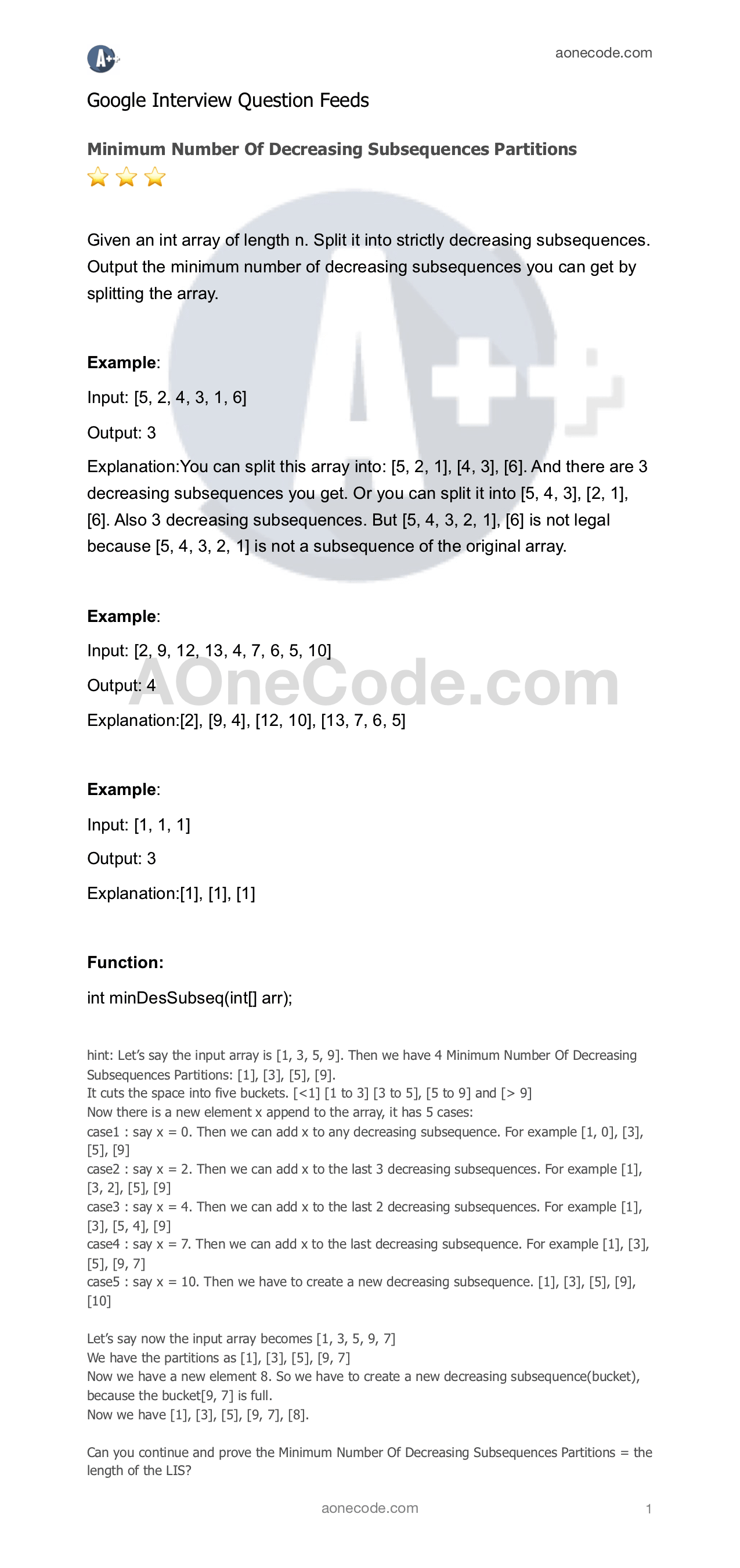#### Rose Garden

Given an array a of roses. For example [1, 2, 4, 9, 3, 4, 1]
a[i] means rose i will bloom on day a[i].
Here the first rose will bloom on day 1, the second rose will bloom on day 2, etc. etc.
Also given an int k, which is the minimum number of adjacent bloom roses required for a bouquet.
and an int n, which is the number of bouquets we need.
return the earliest day that we can get n bouquets of roses.

for example:
a = [1, 2, 4, 9, 3, 4, 1], k = 2, n = 2
day 1:
the first and the last rose bloom.
[b, n, n, n, n, n, b]
day 2:
the second rose bloom
[b, b, n, n, n, n, b]
Here the first two bloom roses make a bouquet.
day 3: [b, b, n, n, b, n, b]
day4: [b, b, n, n, b, b, b]
Here the last three bloom roses make a bouquet, meeting the required n = 2 bouquets of bloom roses.
So return day 4.

#### Fill 2D Array

Given a n*n  2D array. Fill the integer from 1 to n*n to this 2D array that makes the sum of each row, each column and the two diagonal equal.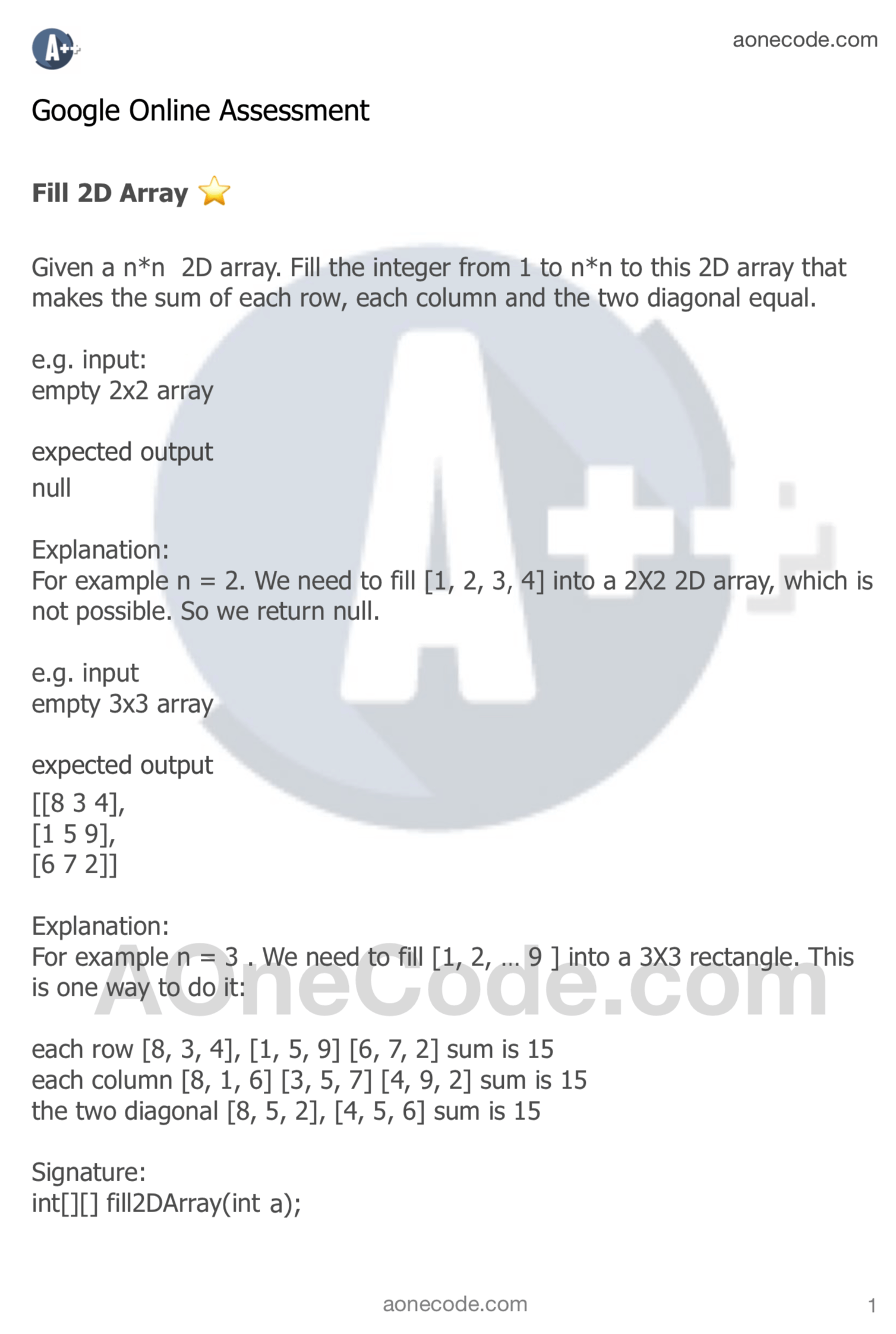#### Google OA : Pick Up Coupons

Given an array of integers representing the value of coupons, return the minimum number of consecutive coupons to pick up to win the prize. Return -1 if it’s not possible...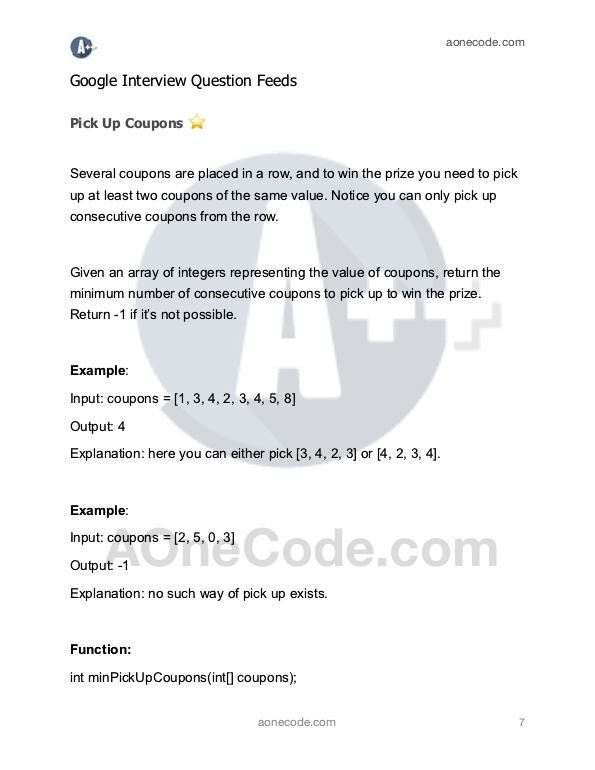#### Google OA : Water Plants

You are a gardener and you take care of your plants. The plants are planted in a row and each of them needs a specific amount of water. You are about to water them using a watering can...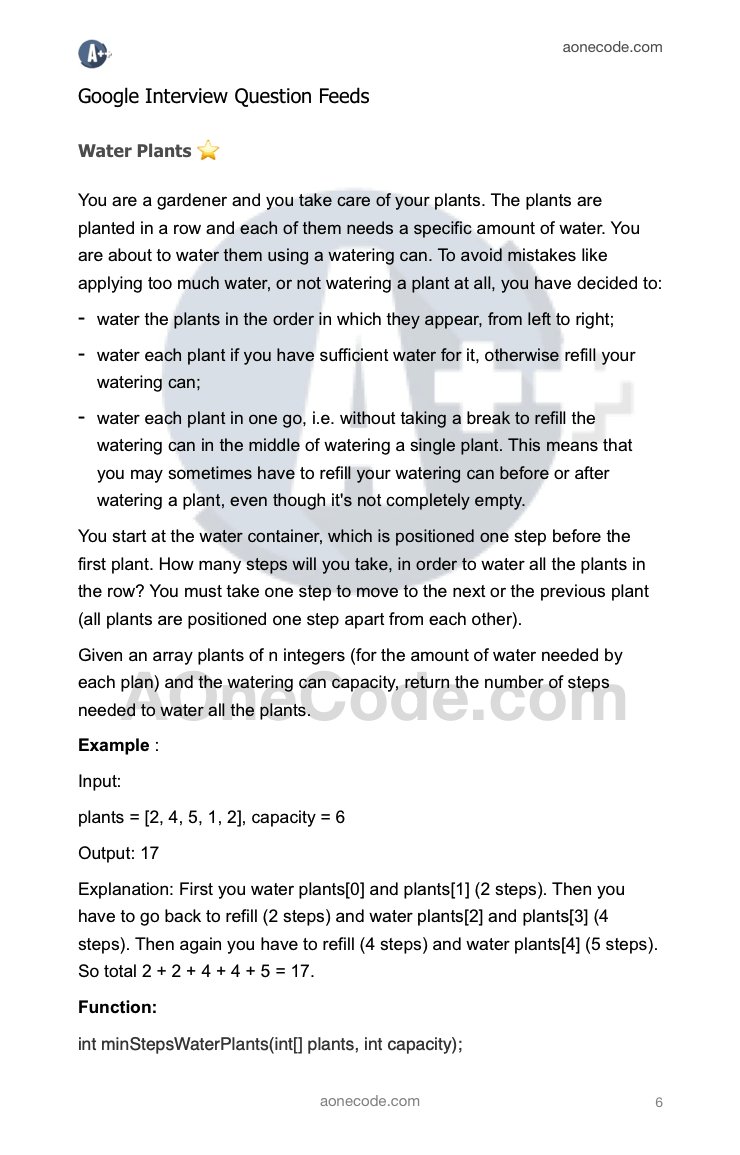#### Google OA : Compare Strings

Write a function that, given string A (which contains M strings delimited by',') and string B (which contains N strings delimited by ','), returns an array C of N integers. For 0 <= J < N, values of C[J] specify the number of strings in A which are strictly smaller than the comparison J-th string in B (starting from 0).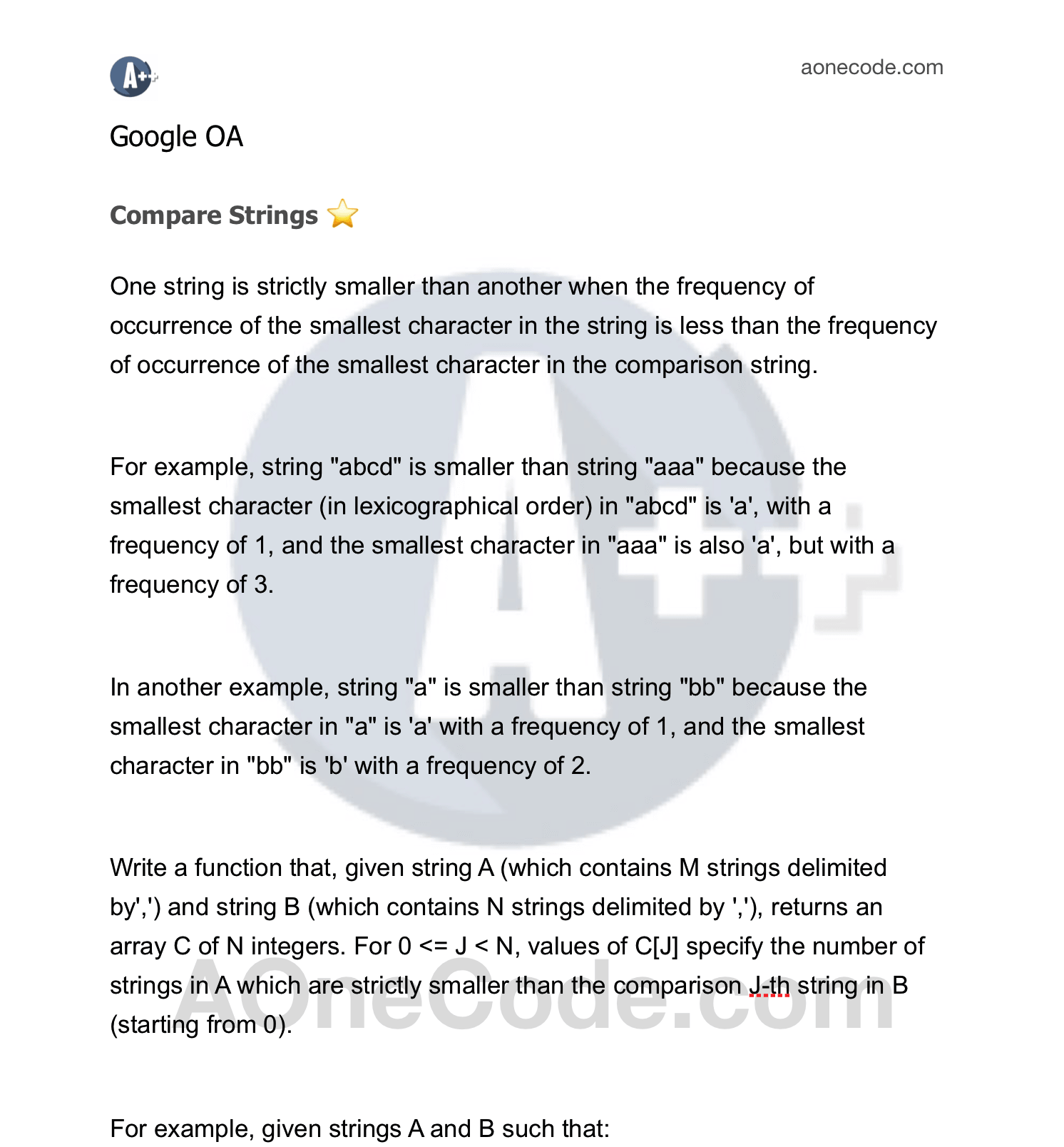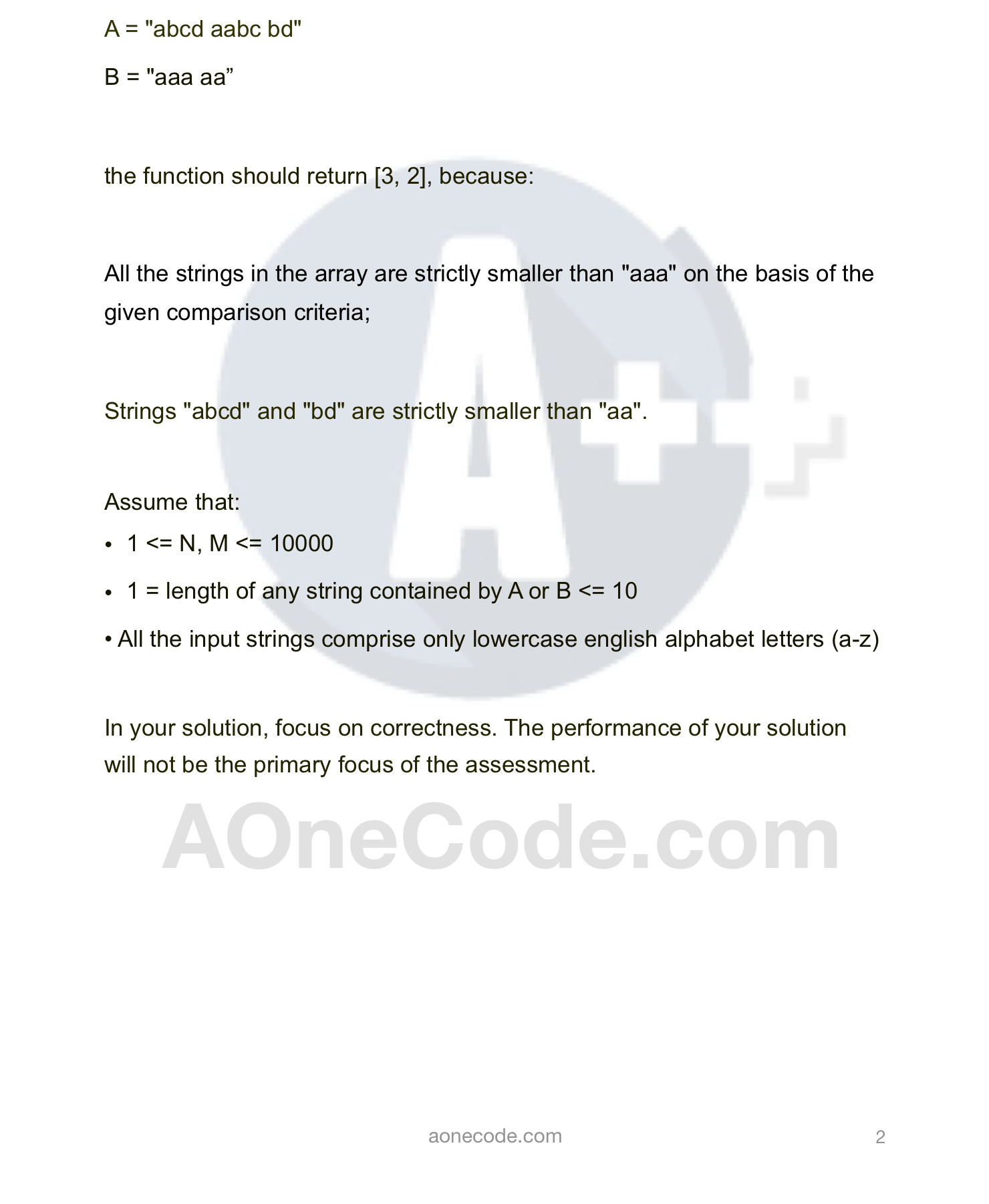#### Google OA : Largest Subarray

Write a function that, given a zero-indexed array A consisting of N integers and an integer K, returns the largest contiguous subarray of length K from all the contiguous subarrays of length K.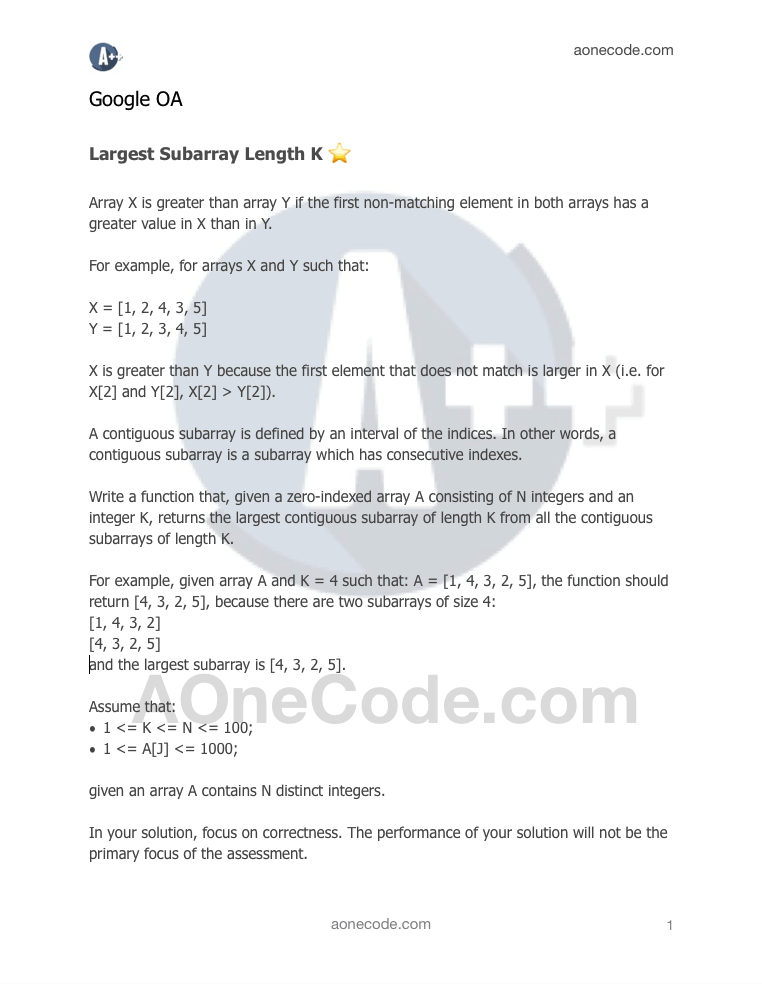#### Maximum Area Serving Cake

Given an array containing the radii of circular cakes and the number of guests, determine the largest piece that can be cut from the cakes such that every guest gets a piece of the cake with the same area. It is not possible that a single piece has some part of one cake and some part of another cake and each guest is served only one piece of cake.

Example 1
Radii = [ 1, 1, 1, 2, 2, 3] numberOfGuests = 6.
Output: 7.0686

Reason being you can take the area of the cake with a radius of 3, and divide by 4. (Area 28.743 / 4 = 7.0686)
Use a similary sized piece from the remaining cakes of radius 2 because total area of cakes with radius 2 are > 7.0686

Example 2
Radii [4, 3, 3] numberOfGuests = 3
Output: 28.2743

Example 3
Radi [6, 7] numberOfGuests = 12
Output: 21.9911

#### Key Formatting

Given a string S consisting of N characters and an integer K. The string represents a software license key which we would like to format. The string is composed of alphanumerical characters and/or dashes. The dashes split the alphanumerical characters within the string into groups (i.e if there are M dashes, the string is split into M+1 groups). The dashes in the given string are possibly misplaced. from aonecode.com

We want each group of characters to be of length K (except for possibly the first group, which could be shorter, but still must contain at least one character). To satisfy this requirement, we will reinsert the dashes. Additionally, all the lower case letters in the string must be converted to upper case. from aonecode.com

For example, in the license key '2-440r7-4k" there are two dashes which split it into three groups of lengths 1, 5 and 2. respectively. if we want each group to be of length 4, then we would have to reinsert the dashes Thus, for K = 4, the correctly formatted string is ''24A0-R74K". from aonecode.com
Write a function:

``````class Solution {
public String solution(String S, int K); from aonecode.com
}
``````

that, given a non-empty string S consisting of N characters. representing a license key to format, and an integer K, returns the license key formatted according to the description above. from aonecode.com

For example, given S = "2-4110r-7-4k" and K = 4, the function should return "2440-R74K", and for K = 3, the function should return "24-40R-74K" as the first group could be shorter. Given S="r" and K = 1, the function should return ''R". from aonecode.com
Hints: O(n) time required. from aonecode.com